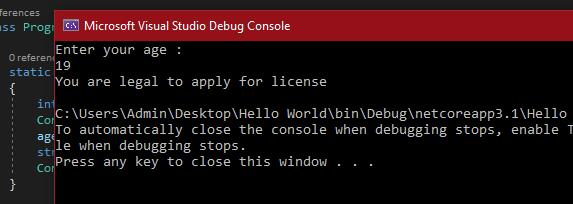Loading, please wait...# Operators in C# | C# Tutorials

Nov 11, 2020 C#, CSharp, 1412 Views
The operators in an object-oriented language have a very crucial role. They are involved in almost every part and working of the program. So in C# we have very much use of these operators too.

## Operators in C# | C# Tutorials

The operators in an object-oriented language have a very crucial role. They are involved in almost every part and working of the program. So in C#, we have very much use of these operators too. They are used for different purposes, such as stating some relation between values, comparing them, making changes to the values like adding, subtracting, and more. This can be used for numeric as well as string values too.

## Pre-requisite

To perform the programs the Visual studio will be used as an integrated development environment. Simply open up the Visual Studio and click on CREATE A NEW PROJECT then select Console App (.Net Core). Name the project then click on Create.

## Understanding Operators

There are different types of operators for different purposes. They all work in their respective manners. The types of operators are:

• Arithmetic Operators
• Relational Operators
• Logical Operators
• Bitwise Operators
• Assignment Operators
• Conditional operators

There is one more thing that in C# language the operators can be categorized, based on the number of operands. That would be:

• Unary Operator: To perform an operation the operator will take one operand.
• Binary Operator: To perform an operation the operator will take two operands.
• Ternary Operator: To perform an operation the operator will take three operands.

### Arithmetic Operators

To carry out mathematical or arithmetical operations in a program the arithmetic operators are used.

There are:

• Addition: in this, the ‘+’ operator adds two operands. For example, a+b.
• Subtraction: in this, the  ‘-‘ operator subtracts two operands. For example, a-b.
• Multiplication: in this, the  ‘*’ operator multiplies two operands. For example, a*b.
• Division: in this the  ‘/’ operator divides the first operand by the second. For example, a/b.
• Modulus: in this the  ‘%’ operator returns the remainder when the first operand is divided by the second. For example, a%b.
``````using System;

namespace Hello_World

{

class Program

{

static void Main(string[] args)

{

int num1, num2;

Console.WriteLine("Insert first Number ");

Console.WriteLine("===============");

Console.WriteLine("Insert second Number ");

Console.WriteLine("===============");

Console.WriteLine("Addition Operator: " + (num1 + num2));

// Subtraction

Console.WriteLine("Subtraction Operator: " + (num1 - num2));

// Multiplication

Console.WriteLine("Multiplication Operator: " + (num1 * num2));

// Division

Console.WriteLine("Division Operator: " + (num1/num2));

// Modulo

Console.WriteLine("Modulo Operator: " + (num1%num2));

}

}

}``````

Output: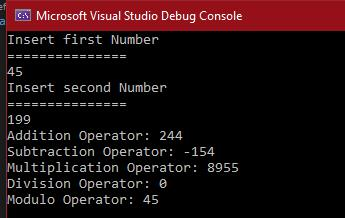In this, there some more things too, such as the precedence and associativity property and also the increment and decrement with prefix and postfix options.

• Precedence Property: This property we have to keep in mind while dealing with more than one operator in a statement, along with the usage of apprentices. With the help of this property, we can decide which operator has to operate first between the operands. There is a short and simple rule for that.
1. ()
2. ^
3. * or /
4. + or –

The above has a top-down approach which means the top most will carry out first then the next and so on.

• Associativity Property: This property is used when we have arithmetic operators of the same level but still different which means * and / at the same time or + and – same time. For this, we put a left to the right approach to sort the priority. For instance
1. * has more priority than /
2. + has more priority than –
• Increment and decrement with postfix and prefix: The ‘++’operator is used to increment the value of an integer. When placed before the variable name it is called the pre-increment operator, it increases the value first. For example, ++a.
And when it is placed after the variable name it is called post-increment operator, it doesn’t increase the value right away but first saves or operates with the value then it increases. For example, b++.

Similarly with decrement of the integer value, but this time the ‘- -‘ operator will be used with the same logic for prefix and postfix.

Example:

``````using System;

namespace Hello_World

{

class Program

{

static void Main(string[] args)

{

int a = 4;

int b = 8;

Console.WriteLine(++a);

Console.WriteLine(a++);

Console.WriteLine(--b);

Console.WriteLine(b--);

}

}

}
``````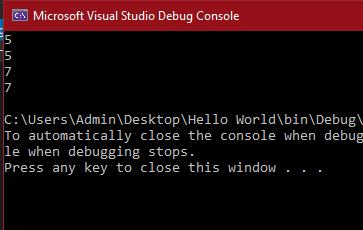### Relational Operators

When it comes to comparing two values which can be numeric or string, the relational operators come in. There are different types of relational operators:

• Equal to “==”: This operator checks for whether two operands are equal or not.
• Not Equal to “!=”: This operator checks for whether two operands are not equal or not.
• Grater than “>” and Less than “<”: These are used to compare which one of the operands is greater or smaller than the other.
• Greater than equal to “>=” and Less than equal to “<=”: These are used to set a greater or lesser limit to an operand as a limit. This means if it says all numbers from 1 to less than equal to “<=” 5 then all the numbers from 1 to 5 will be included.

They are widely used in looping actually and many conditional rendering statements such as if and if-else.

Example:

``````using System;

namespace Hello_World

{

class Program

{

static void Main(string[] args)

{

int a = 5;

int b = 8;

for (int i = 0; i <10; i += 2)

{

if(a < b)

{

Console.WriteLine("a is less than b");

}else if( a == b)

{

Console.WriteLine("a is equal to b");

}

else

{

Console.WriteLine("a is greater than b");

}

a++;

}

}

}

}``````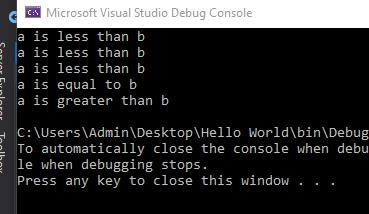### Logical Operators

When there is a need to compare two conditions in a statement, we need logical operators. There are only a few logical operators such as:

• Logical AND (&&): This is like if either one is false whole is false.
• Logical OR (||): This is like if either one is true, while is true.
• Logical NOT (!): This just gets the negative/opposite result, which means it will make true to false and vice versa.

NOTE: It returns answers/values in terms of true or false only.

Example:

``````using System;

namespace Hello_World

{

class Program

{

static void Main(string[] args)

{

bool a = true, b = false, result;

result = a && b;

Console.WriteLine("AND Operator: " + result);

result = a || b;

Console.WriteLine("OR Operator: " + result);

result = !a;

Console.WriteLine("NOT Operator: " + result);

}

}

}``````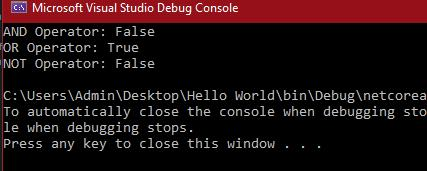### Bitwise Operators

There are 6 bitwise operators in C#. They are used to operates on a bit string, a bit array, or a binary numeral at the level of its individual bits.

The operators are:

1. AND “&”
2. OR “|”
3. XOR “^”
4. Compliment “~”
5. Left Shift “<<”
6. Right shift “>>”

To understand them better take a look at the code and its output.

Example:

``````using System;

namespace Hello_World

{

class Program

{

static void Main(string[] args)

{

int x =12 , y = 111, result;

// Bitwise AND Operator

result = x & y;

Console.WriteLine("Bitwise AND: " + result);

// Bitwise OR Operator

result = x | y;

Console.WriteLine("Bitwise OR: " + result);

// Bitwise XOR Operator

result = x ^ y;

Console.WriteLine("Bitwise XOR: " + result);

// Bitwise AND Operator

result = ~x;

Console.WriteLine("Bitwise Complement: " + result);

// Bitwise LEFT SHIFT Operator

result = x << 2;

Console.WriteLine("Bitwise Left Shift: " + result);

// Bitwise RIGHT SHIFT Operator

result = x >> 2;

Console.WriteLine("Bitwise Right Shift: " + result);

}

}

}``````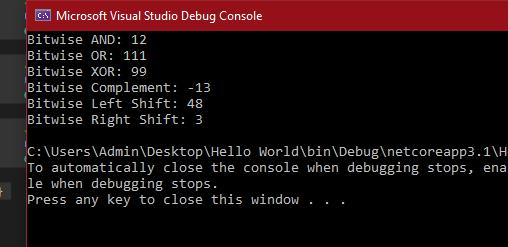### Assignment Operators

The assignment operators are generally used for assigning some value to a variable. They follow a specific pattern which is, the value will be assigned to the left operand. The value on the right side must be of the same data-type as the variable on the left side otherwise the compiler will raise an error.

Some of the assignment operators are:

• Simple assignment: In this “=” operator is used, which simply assigns the value of the right operand to the left operand.

Example:

A = 10;

B = 15;

Wr = “abc”;

• Add assignment: In this “+=” operator is used. This adds up the right operand to the left operand. Then assign the final value to the left operand.

Example:

A += b which means A = A + B

• Subtract assignment: In this “-=” operator is used. This subtracts up the right operand to the left operand. Then assign the final value to the left operand.

Example:

A -= b which means A = A - B

• Multiply assignment: In this “*=” operator is used. This multiplies up the right operand to the left operand. Then assign the final value to the left operand.

Example:

A *= b which means A = A * B

• Division assignment: In this “/=” operator is used. This divides up the left operand with the right operand. Then assign the final value to the left operand.

Example:

A /= b which means A = A / B

There are more of them, plus they are widely used in almost every program.

### Conditional Operator

The other name of this is the Ternary Operator. This is just like a different version of writing the if and else statement. It returns any of the two values depending on the Boolean expression. That is if the condition is true it will return the first statement or else the second.

Syntax:

``condition ? first_expression : second_expression;``

Example:

``````using System;

namespace Hello_World

{

class Program

{

static void Main(string[] args)

{

int age;